Tamilnadu State Board New Syllabus Samacheer Kalvi 12th Maths Guide Pdf Chapter 1 Applications of Matrices and Determinants Ex 1.2 Textbook Questions and Answers, Notes.

## Tamilnadu Samacheer Kalvi 12th Maths Solutions Chapter 1 Applications of Matrices and Determinants Ex 1.2

Question 1.
Find the rank of the following matrices by minor method:Solution:

(i) A = $$\begin{bmatrix} 2 & -4 \\ -1 & 2 \end{bmatrix}$$
A is a matrix of order 2 × 2 and p(A) ≤ 2
Second order minor
|A| = $$\begin{bmatrix} 2 & -4 \\ -1 & 2 \end{bmatrix}$$
= 4 – 4 = 0
∴p(A) ≠ 2
First order minor is non vanishing
p(A) = 1

(ii) A = $$\left[\begin{array}{rr} -1 & 3 \\ 4 & -7 \\ 3 & -4 \end{array}\right]$$
A is a matrix of order 3 × 2 and p(A) ≤ 2
Second order minor
$$\begin{bmatrix} -1 & 3 \\ 4 & -7 \end{bmatrix}$$
= 7 – 12 = -5 ≠ 0
∴ p(A) = 2

(iii) A = $$\left[\begin{array}{rrrr} 1 & -2 & -1 & 0 \\ 3 & -6 & -3 & 1 \end{array}\right]$$
A is a matrix of order 2 × 4 and p(A) ≤ 2
Second order minor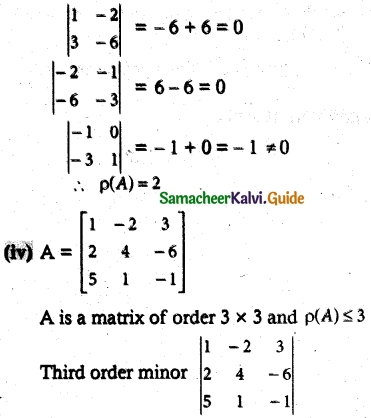= 1(-4 + 6) + 2(-2 + 30) + 3(2 – 20)
= 2 + 56 – 54 = 4 ≠ 0
∴p(A) = 3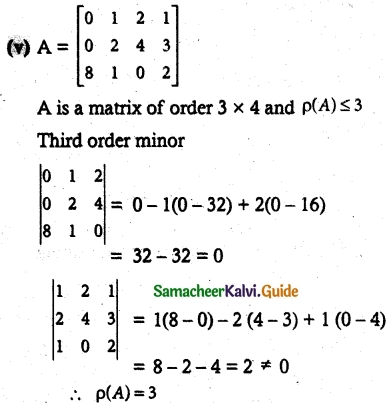Question 2.
Find the rank of the following matrices by row reduction method: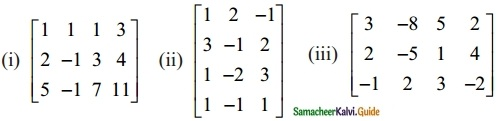Solution:The last equivalent matrix is in row echelon form. It has two non-zero rows.
∴ p(A) = 2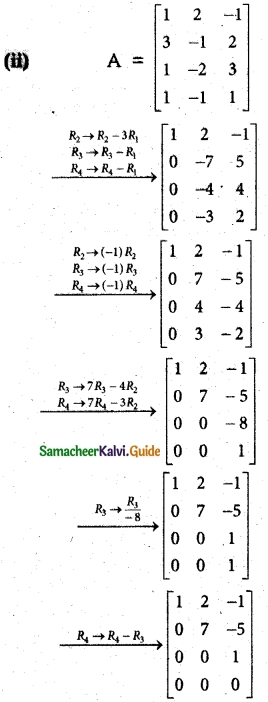The last equivalent matrix is in row echelon form. It has three non-zero rows.
∴ p(A) = 3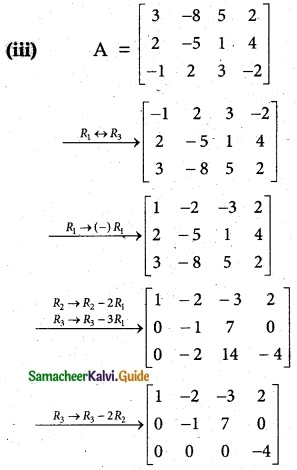The last equivalent matrix is in row echelon form. It has three non-zero rows.
∴ p(A) = 3Question 3.
Find the inverse of each of the following by Gauss-Jordan method: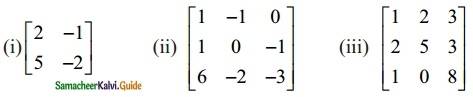Solution: